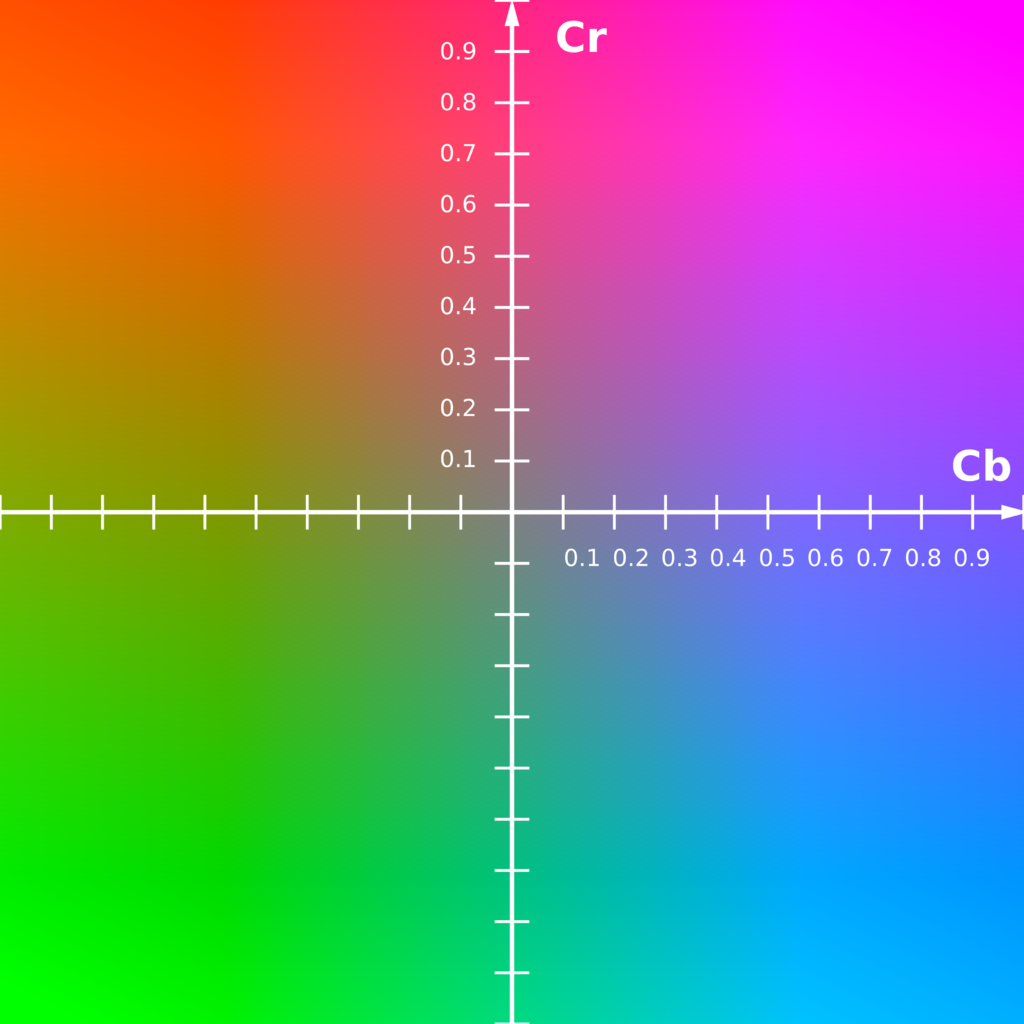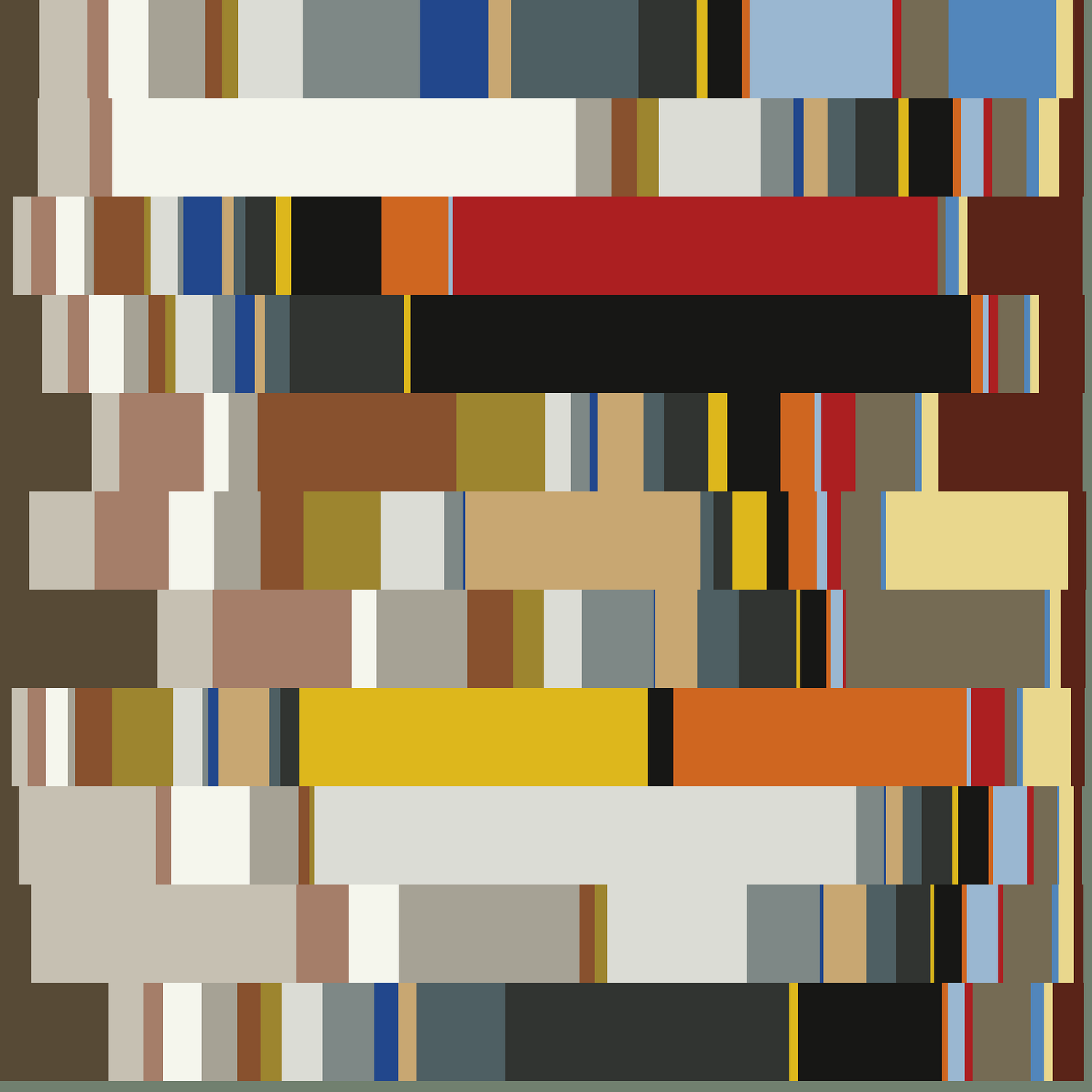# Extracting Colour Palettes with Unsupervised Learning

## Color combination analysis with chained clustering

For a recent project I needed to find a method to determine which colour palettes were present in a large dataset of artworks, ideally without any input from myself! This task aligned itself to the unsupervised technique of clustering and can be divided into two steps:

1. Extracting commonly occurring colours.
2. Extracting common combinations of these colours.

Both of these can be accomplished with K-Means clustering, which simply clusters points by the distance between points and cluster centers.

# 1. Extracting commonly used colours.

To effectively use K-means to extract colour combinations it is important to have the euclidean distance between colour data points be interpretable as perceived difference in colours. Simply using the red-green-blue (RGB) channels of the images as a three dimensional vector does a rather poor job of this, as distance in RGB space does not translate well into perceived difference.

To overcome this the image is converted into YCbCr colour space, with the Y, Cb, Cr channels forming three-dimensional vectors to represent each color. Distances in this colour space do a much better job at representing differences in perceived colour.YCbCr Colour Space, the Y axis runs into the screen and represents lightness

After converting the loaded dataset into YCbCr and extracting a large random sample of pixels, K-means with n clusters will extract n commonly used colours as the cluster centers. As these centres mark the average of the colour clusters, the colours produced can be rather muted if an insufficient number of clusters are used. Empirically I found that 20 to 40 clusters produced good results.

# 2. Extracting common combinations of these colours.

Once the common colours have been found, we want to find out in what combination and proportions these colours are commonly used.

This can be done by taking a fixed sample of pixels from each image and seeing which colour cluster each pixel belongs to. These groupings can be aggregated as a count of each colour by image and stored as an n-dimensional vector that represents the colour usage in each image. Using counts in this manner captures the proportion as well as presence of each colour. As all of these image vectors have the same sum, these image representations lie on the hyperplane that intersects all axes at the size of the pixel sample.

K-means can then be applied to these count vectors to extract the common combinations and proportions of the colours found before, with m-cluster centres yielding m different colour palettes.Example of m=11 colour palettes with n=22 different colours. Original Graphic

This system can be tested by providing a dataset that represents images from two very different colour pallete distributions — bright rainbows and earthy desserts. Setting the number of colours at a conservative 15 and the number of colour pallets to two we can see how the different palettes are extracted through the two steps.